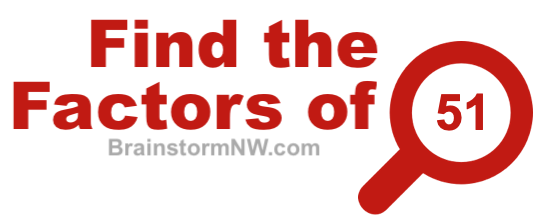Find the factors of 51Do you know all the factors of 51? Use our factors of 51 puzzle below to see how well you know the factors of 51.

There are 4 factors of 51. Below you see one hundred numbers. If you click on a number that is a factor of 51, then it will turn green. If you click on a number that is not a factor of 51, then it will turn red.

See if you can get 4 green squares and no red squares. You can see the status of your progress below the numbers further down on the page.

Are you a student? Did you find all the factors of 51 above or did you only find some of the factors of 51? Check out Factors of 51 if you need to brush up on factorization and how to find the factors of 51.

Find the Factors
Do you want to play "Find the Factors" for another number? If so, please enter the number below and press Play!

Find the factors of 52
Here is another factor puzzle you may find entertaining and educational.

Copyright  |   Privacy Policy  |   Disclaimer  |   Contact